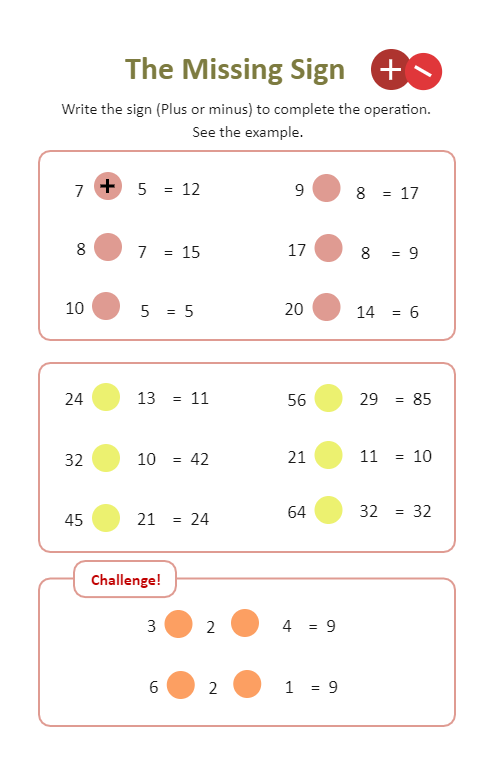Template Community / Addition and Subtraction WorksheetBlackwood
Published on 2022-03-03
Edit OnlineTeaching addition and subtraction are the two most important mathematical solutions. By teaching your students about addition and subtraction, you are introducing them to the world of algebra, geometry, trigonometry, and some advanced mathematics like differentiation, calculus, algorithms, and more. In the following addition & subtraction worksheet, the task is to find the missing sign. We have mentioned the number, and the students must identify the missing sign. For instance, we have 8 _ 7 = 15. The student will have to think and decide which mathematical sign (+ or -) they will have to mention in the blank space. Similarly, the student can fill out the remaining empty spaces as well.
Tag
science diagram
Mathematics Diagram
Report
0
54PostRecommended Templates